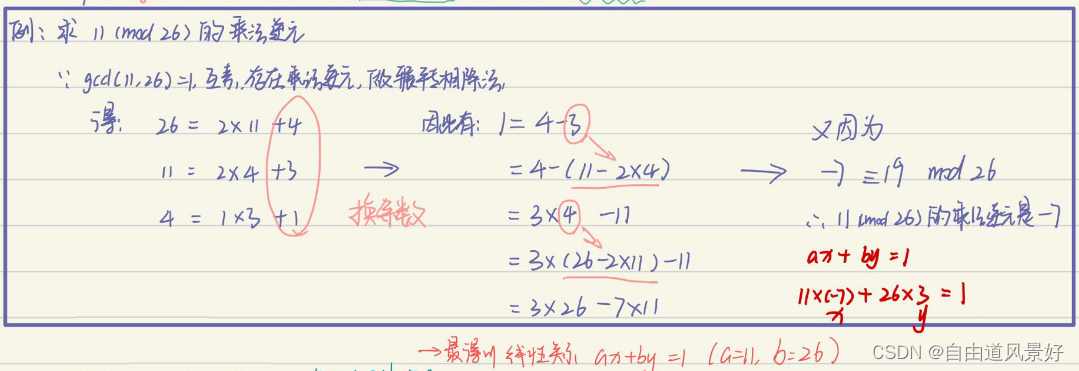• 模逆运算怎么求
万次阅读
2018-01-01 22:45:55

模逆的定义：

要定义这个运算，需要三个整数。a的模逆元素（对n取模）为b，意味着a*b mod m=1，则称a关于m的模逆为b


Python实现:

1.
def     gcd(a,b):
while a!=0:
a,b = b%a,a
return b
#定义一个函数，参数分别为a,n，返回值为b
def     findModReverse(a,m):#这个扩展欧几里得算法求模逆

if gcd(a,m)!=1:
return None
u1,u2,u3 = 1,0,a
v1,v2,v3 = 0,1,m
while v3!=0:
q = u3//v3
v1,v2,v3,u1,u2,u3 = (u1-q*v1),(u2-q*v2),(u3-q*v3),v1,v2,v3
return u1%m
2.
def     findModReverse(a,m):用定义求，但是效率很低
import itertools
for b in itertools.count(1):
if (a*b)%m==1:
return bpython 算法 扩展
更多相关内容
• 因此寻找乘法逆元的过程就是x和y的过程。 这里我们先看一下人工计算的过程： 具体实现时使用三组变量：x1，x2，x3；y1，y2，y3；t1，t2，t3。初始化时，给x1，x2，x3别赋值1、0、a，则有 1 × a + 0 × b = a ...

扩展欧几里得算法

给予二整数 a 与 b, 必存在有整数 x 与 y 使得  ax + by = gcd(a,b)

有两个数a,b，对它们进行辗转相除法，可得它们的最大公约数——这是众所周知的。然后，收集辗转相除法中产生的式子，倒回去，可以得到ax+by=gcd(a,b)的整数解。

### 1、算法原理

给定整数a、b，若gcd(a,b)=1。则存在c，满足ac=1 mod b，c即为a模b的乘法逆元。

利用扩展的欧几里得算法求得满足条件的c：先做辗转相除，当a、b互素时，最后一步得到的余数为1，再从1出发，对前面得到的所有除法算式进行变形，将余数用除数和被除数表示，最终便可将1表示为a与b的一种线性组合，即

从而x就是a模b的乘法逆元。因此寻找乘法逆元的过程就是求x和y的过程。

这里我们先看一下人工计算的过程：具体实现时使用三组变量：x1，x2，x3；y1，y2，y3；t1，t2，t3。初始化时，给x1，x2，x3别赋值1、0、a，则有

1 × a + 0 × b = a

类似地，给y1，y2，y3分别赋值0、1、b，有

0 × a + 1 × b = b

接下来开始迭代，保证在每次迭代中，有

a × t1 + b × t2 = t3

成立。为此只需在每一步中为 ti 分别赋值  (i=1,2,3) ，其中q为x3除以y3的商。

当最后t3迭代至计算结果为1时，相应的 t1 就是 a模b的乘法速元，而 t2 是b模a的乘法逆元。

### 2、代码部分

#include <stdio.h>
int main(int argc, char *argv[])
{
int temp,q,t1,t2,t3;
int a,b,swap=0;
int x1,x2,x3,y1,y2,y3;
printf("Input two integers: ");
scanf("%d",&a);
scanf("%d",&b);
if (a<b) {   //保证a>b
swap = 1;
temp = a;
a = b;
b = temp;
}
x1=1;	x2=0;	x3=a;    // 初始
y1=0;	y2=1;	y3=b;
while (y3!=0)
{
q=x3/y3; //商
t1=x1-q*y1;	t2=x2-q*y2;	t3=x3-q*y3;
x1=y1;		x2=y2;		x3=y3;
y1=t1;		y2=t2;		y3=t3;
printf("\nt1,t2,t3\t%d\t%d\t%d ;",t1,t2,t3);
}
printf("\n");
if(x3 == 1)   // 这里使用x3，组后一轮循环中，x3保存了上一步中值为1的t3
{
if(swap==1)
{
printf("\ninverse of %d mod %d is:%d",b,a,x2);
printf("\ninverse of %d mod %d is:%d",a,b,x1);
}
else{
printf("\ninverse of %d mod %d is:%d",a,b,x2);
printf("\ninverse of %d mod %d is:%d",b,a,x1);
}
}
else  printf("no inverse");
printf("\n\n\n");
return 0;
}

注释：

关于判断条件是  x3==1，而不是 t3==1 的问题，while循环中本质使用的是辗转相除法，对于 ti 的赋值表达式，先看t3，t3=x3-q*y3 ，q=x3/y3 ，我理解的是 t3 就是 x3除以y3的余数，加上开始时,给 x3=a，y3=b ，所以对t3的迭代可以看成对 （a,b）做辗转相除法。

再看 t1 和 t2，为了保持线性关系ax + by = gcd(a,b)，相应的x和y的值也需要变化，这就是 t1 和 t2 的作用。

展开全文c++ 算法 c语言
• ## 模逆运算（C语言）

千次阅读 2020-03-06 13:04:26
模逆运算（C语言） 简介 使用扩展欧几里得算法 代码实现 #include <stdio.h> int main() { int temp,q,t1,t2,t3,i=1; int a,b,swap=0; int x1,x2,x3,y1,y2,y3; /**欢迎**/ printf("--------欢迎使用模逆...

## 模逆运算（C语言）

简介

使用扩展欧几里得算法

代码实现

#include <stdio.h>

int main()
{
int temp,q,t1,t2,t3,i=1;
int a,b,swap=0;
int x1,x2,x3,y1,y2,y3;
/**欢迎**/
printf("--------欢迎使用模逆运算-----------\n");
printf("请输入两个整数，以空格分开\n");
scanf("%d",&a);
scanf("%d",&b);
if(a<b){  //交换a，b
swap = 1;
temp = a;
a = b;
b = temp;
}
x1=1;x2=0,x3=a;
y1=0;y2=1,y3=b;
while(y3 != 0){
q = x3/y3;
t1=x1-q*y1;t2=x2-q*y2;t3=x3-q*y3;
x1=y1;x2=y2;x3=y3;
y1=t1;y2=t2;y3=t3;
printf("第%d次迭代，t1=%d,t2=%d,t3=%d\n",i,t1,t2,t3);  //打印迭代的值
i++;
}
if(x3 == 1){
if(swap == 1){
printf("模逆运算：%d mod %d 为：%d\n",b,a,x2);
printf("模逆运算：%d mod %d 为：%d\n",a,b,x1);
} else{
printf("模逆运算：%d mod %d 为：%d\n",a,b,x2);
printf("模逆运算：%d mod %d 为：%d\n",b,a,x1);
}
} else{
printf("无法进行模逆运算！");
}
return 0;
}

展开全文• 信息安全数学基础--同余方程--同余方程运算：模逆运算，模指数运算 博主本人是初学信息安全数学基础（整除+同余+原根+群环域），本意是想整理一些较难理解的定理、算法，加深记忆也方便日后查找；如果有错，欢迎指正...

### 初等数论--同余方程--同余方程运算：模逆运算，模指数运算

博主是初学初等数论（整除+同余+原根），本意是想整理一些较难理解的定理、算法，加深记忆也方便日后查找；如果有错，欢迎指正。
我整理成一个系列：初等数论，方便检索。

同余方程： a x ≡ b ( m o d m ) ax\equiv b(mod m)

• 模逆运算：若 ( a , m ) = 1 , (a,m)=1, 那么由上一章信息安全数学基础–同余方程–同余方程的解证明的“同余方程 a x + b ≡ 0 ( m o d m ) ax+b\equiv 0(mod m) 的解个数为 ( a , m ) (a,m) ”得，同余方程 a x ≡ b ( m o d m ) ax\equiv b(mod m) 的解个数为 ( a , m ) (a,m)
a x ≡ b → x ≡ a − 1 b ( m o d m ) ax\equiv b\rightarrow x\equiv a^{-1}b(mod m)
进行模逆运算，求 a − 1 ( m o d m ) a^{-1}(mod m) ,即求方程 a x ≡ 1 ( m o d m ) ax\equiv 1(mod m) 的解, → a x = 1 + k m → a x − k m = 1 ↔ a x + m y = 1 \rightarrow ax=1+km \rightarrow ax-km=1\leftrightarrow ax+my=1 ，运用欧几里得算法/辗转相除法 求 x x

• 模指数运算：若 ( a , m ) = 1 , (a,m)=1, 那么由欧拉定理知 a φ ( m ) ≡ 1 ( m o d m ) a^{\varphi(m)}\equiv 1(mod m) ,那么 a x ≡ b → x ≡ a − 1 b → x ≡ a φ ( m ) − 1 b ( m o d m ) ax\equiv b\rightarrow x\equiv a^{-1}b\rightarrow x\equiv a^{{\varphi(m)}-1}b(mod m)
进行模指数运算，求 a n ( m o d m ) a^n(mod m)
n = n k ⋅ 2 k + n k − 1 ⋅ 2 k − 1 + … … + n 1 ⋅ 2 1 + n 0 ⋅ 2 0 a n = a n k ⋅ 2 k + n k − 1 ⋅ 2 k − 1 + … … + n 1 ⋅ 2 1 + n 0 ⋅ 2 0 = ( a n k ) 2 ⋅ a n k − 1 ) 2 … … a n 1 ) 2 ⋅ a n 0 n=n_k·2^k+n_{k-1}·2^{k-1}+……+n_1·2^1+n_0·2^0\\ a^n=a^{n_k·2^k+n_{k-1}·2^{k-1}+……+n_1·2^1+n_0·2^0}\\ =(a^{n_k})^2·a^{n_{k-1}})^2……a^{n_1})^2·a^{n_0}

展开全文数学
• ## c/c++实现模逆运算

千次阅读 2020-03-06 10:50:05
c/c++实现模逆运算 最终结果: 实验原理： 代码实践： #include <iostream> using namespace std; int main(int age, char * argv[]){ int temp, q, t1, t2, t3; int a, b, swap=0; int x1, x2, x3, y1,...算法 c++
•python 编程语言 脚本语言
• 举例说明：7的模26（n）的逆 26 = 3（a） * 7 +5（b）  7 = 1 *5 +2  5 =2 * 2 +1 2 = 1*2 +0 把对于每一行式子的乘数a（余数为0 的除外），从后往前排列,如下  2 1 3 （I） 1 2 3 11（final） ...
• 模幂运算public static int modPowerShow(int a, int k, int n){System.out.println("*************************************************************************");System.out.println("****NOTICE:[\"\\\" area ...
• 本发明涉及模逆计算技术领域，更具体地说，涉及一种模逆运算方法及运算器。背景技术：模逆运算广泛应用在公钥密码体制中，例如，在RSA算法中的解密密钥生成时应用到模逆运算模逆运算也可以用于椭圆曲线密码算法中...
• 一道Matlab编程题 &暴力解法 Matlab课上老师出了这样一道题： 一个篮子有K个鸡蛋： 2个2个拿剩1个；...篮子里鸡蛋的个数K 虽然这是一道matlab拿来玩的题目，可是我觉得完全...
• miracl中有许多可以乘法逆元的函数，在这里主要介绍函数xgcd()1.函数介绍2.涵盖内容3.注意事项一、xgcd：函数原型：int xgcd(x,y,xd,yd,z)big x,y,xd,yd,z;在大数库中，xgcd的计算公式是：On exit z=gcd(x,y)=x.xd...
• 拿到题目 一串加密密文 fR4aHWwuFCYYVydFRxMqHhhCKBseH1dbFygrRxIWJ1UYFhotFjA= 下载附件之后拿到源码 <?php function encrypt($data,$key) { $key = md5('ISCC');$x = 0;... $klen = strlen($k...
• } 然后我们进行模逆操作 //模逆运算，用模逆算法，此处编写程序时候u,v为实际计算过程中v的各个值 int mod_invese(int d,int n)//d模n的逆，n 为正整数 { int a,b,q,r,u=0,v=1,t;//a为被除数，b为除数，q为商 a...c语言
• 介绍 例如 [[1,2],[3,4]] mod 7 = [[5,1],[5,3]] (mod 7) Sage (www.sagemath.org) as Matrix(IntegerModRing(7), [[1, 2], [3,4]]).inverse() python代码 import numpy ... nc = vmnp.shapepython
• java.math.BigInteger.modInverse(BigInteger m)返回一个BigInteger，其值为(this-1 mod m)。使用这种方法，您可以计算给定数字的模乘逆。程序importjava.math.*;publicclassBigIntegerDemo{publicstaticvoidmain...
• 运算与基本四则运算有些相似，但是除法例外。其规则如下： (a + b) % p = (a % p + b % p) % p (a - b) % p = (a % p - b % p) % p (a * b) % p = (a % p * b % p) % p (a^b) % p = ((a % p)^b) % p 推论： 若a≡b...
• SM2公钥密码在智能卡领域有广泛的应用，其运算中难以避免模逆运算，而模逆算法因为其具有幂指数级别的运算复杂度，成为制约SM2算法性能的一个重要瓶颈。以SM2算法公钥引擎为基础，巧妙地利用了已有的蒙哥马利乘法器...
• 欧几里得算法也称碾转相除法，是目前已知最大公约数得最快通用方法，具有代码复杂度低、易理解、用途广众多优点。
• 但是你会发现费马小定理和扩展欧几里得算法逆元是有局限性的，它们都会要求与互素。实际上我们还有一种通用的逆元方法，适合所有情况。公式如下: 证明步骤如下: 关于逆元的题目 参考代码： #include #include #...
• 简单讲解用辗转相除法计算乘法逆元，用于密码学加密，附C语言实现算法（对正整数运算...